# The Ideal Gas Law PV=nRT

Back to Gas Laws

### The Ideal Gas Law

The ideal gas law can be seen as a combination of all four other gas laws, Charles' Law, Boyle's Law, Gay-Lussac's Law and Avogadro's Law. This law applies to a hypothetical gas called an Ideal Gas. An Ideal Gas is a gas that meets all of the postulates of kinetic molecular theory shown on the previous page. It should be noted that this is a serious limitation. No real gas behaves like an ideal gas, because real atoms do interact with each other somewhat, and no atom has zero size. It is true, however, that as the pressure gets lower and temperature gets higher a real gas does approximate an ideal gas. Because gase particles under these conditions are far apart and moving fast, interactions between gas particles is low.

The Ideal Gas Law It is generally written like this: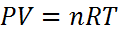Equation #1

The one new thing you haven't seen in the other laws is a new letter, "R". This letter stands for something called the Ideal Gas Constant. All of the other gas laws had constants, but they varied depending on the units you were using as well as the initial conditions. This constant only varies based on the units you are using, and you can look up its value for almost and conceivable set of units. Since we almost always use liters and Kelvin for volume and temperature, we only need to worry about pressure.

The most common value that we will use is the first of the following three:

 pressure in atm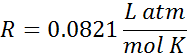pressure in mmHg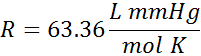pressure in kPa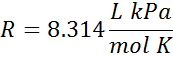The units for volume and pressure are on the top, and the units for moles and temperature are on the bottom. We would say the first one like 0.0821 Liter Atomospheres per mole Kelvin (or per Kelvin mole). The units are also sometimes written with negative superscripts rather than as a fraction. When written this way the first value in the table looks like, R = 0.0821 L atm mol-1 K-1.

It is easy to solve the Ideal Gas Law for any variable, since the equation is in a straight line. Just do the same thing that you do for the combined gas law. Move everything you don't want on one side to the bottom of the other side..

For example: Solving for T is easy.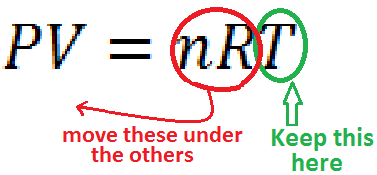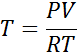Just circle the one you want to keep, and move the others underneath the other side Presto - You're done

All you have to do now is substitute your known variables to get the unknown, T. Remember that units for temperature, T, are in Kelvin, not °C, so you must convert if necessary.

#### Practice

Click on the image above to go go to a site where you can practice Ideal Gas Law probems in an infinite number of combinations

### Development of the IDEAL Gas Law

The combined gas law can be seen as a combination of all four common gas laws, Charles' Law, Boyle's Law, the Gay-Lussac's Law, and Avogadro's Law. It was first proposed in the 1834 by Émile Clapeyron, and later independently derived from kinetic theory by August Krönig in 1856 and Rudolf Clausius in 1857.

The acutal value for R, the ideal gas constant, is a combination of Avogadro's constant and the Boltzman contant. It can be derived to fit what ever units are required. For example - values exist for both versions of absolute temperature °R and Kelvin, volumes as different as cubic feet and milliliters, pressures such as psi, atm, Bar, torr, or kPA, and even different values for moles, such as gram-moles and pound-moles. Derivation in other units is quite easy, actually. If you already have one value, all you need is to apply multiple unit conversions to get R in other units.#### Worksheets

Here are some worksheets with problems about the Ideal Gas Laws.#### PowerPoints

Here you can find some PowerPoints covering the Ideal Gas Laws.#### External Videos

There are a few sources of information about gas laws on the internet. Here are a few of them